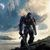# New to Qlik Sense

If you’re new to Qlik Sense, start with this Discussion Board and get up-to-speed quickly.

Announcements
Skip the ticket, Chat with Qlik Support instead for instant assistance.
cancel
Showing results for
Did you mean:Partner - Contributor II

## Line chart with values >0

I have a table that contains several transactions on each combination month+project and I want to create a line chart in Qlik Sense with Dim: Month.

So, problems:
1. Each month in the diagram should show the total amount for the month but only include projects where the total amount for the period is >0. Projects where total amount for the period <0 should not be included in the diagram.
2. I want the values to be accumulated (add the values for each month).

I have tried like this for problem 1. But, you can't put the if-statement outside because then I will get the wrong result:
If(
Sum(
aggr(
Sum({<Year = {\$(#=max(Year))}, Month= } >} Price)
,Month,  Project))

> 0,

Sum(
aggr(
Sum({<Year = {\$(#=max(Year))}, Month= >} Price)
,Month,  Project))

, null() )

Labels (4)

• ### Set AnalysisSpecialist III

Hi,

1. As a calculated dimension while suppressing Null/Zero Value

=Aggr( If ( Sum({<Year = {\$(#=max(Year))}, Month= } >} Price) > 0, Month), Month, Project)

2. =If(RowNo()=1,Sum({<Year = {\$(#=max(Year))}, Month= } >} Price) ,Sum({<Year = {\$(#=max(Year))}, Month= } >} Price) +Above(Expressionlabel,1))Tags
Community Browser